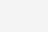# 单片机控制字符型lcd1602显示案例

51单片机驱动 LCD1602液晶显示系统 PROTEUS仿真及源代码设计

LCD1602 51单片机最小系统应用仿真及制作/*************************功能************************/

/*使用外部中断INTO改变LED状态，并且用1602显示出其状态*/

#include<reg51.h>

#include <intrins.h>

#define uint unsigned int

#define uchar unsigned char

uchar code table[]=” HELLO 1602 “;

uchar code table0[]=”1602 TEST “;

uchar code table1[]=”RESULT: “; //空格是写到LCD了，即空格也是内容

uchar code table2[]=”OK!”;

uchar code table3[]=”liang”;

uchar code table4[]=”mie”;

uchar num;

sbit led=P1^7;

sbit lcden=P2^0; //液晶使能端

sbit lcdrs=P2^2; //液晶数据命令选择端选择写数据还是写命令

void delay(uint z) // 延时函数

{

uint i,j;

for(i=z;i>0;i–)

for(j=110;j>0;j–);

}

void write_com(uchar com) //写入

{

lcdrs=0; //LCD 选择输入命令

P0=com; //向P0 口输入命令 39-32端口

delay(5); //延时

lcden=1; //打开LCD 使能

delay(5); //一个高脉冲

lcden=0; //关闭LCD 使能

}

void write_data(uchar date)

{

lcdrs=1; //设置为输入数据

P0=date; //将数据赋给P0 口

delay(5); //延时

lcden=1; //置高

delay(5); //高脉冲

lcden=0; //置低完成高脉冲

}

void init() //LED 初始化

{

lcden=0;

write_com(0x38);//设置16×2 显示5×7 点阵，8 位数据接口

write_com(0x0c); //设置开始显示不显示光标 （指令4）

write_com(0x06); //写一个字符后地址指针加1 （指令3）

write_com(0x01); //显示清零数据指针清零 （指令1）

}

void display(unsigned char *p)//按指针 地址写入数据

{

while(*p!=’0′)

{

write_data(*p); //写数据

p ;

delay(1);

}

}

void main()

{

TCON=0x01; //设置中断为INTO

IE=0x81;

led=0;

init();

write_com(0x80); //将数据指针第一行第一个字处，

display(table); //调用函数的方式写入数据

delay(800);

write_com(0x80); //将数据指针第一行第一个字处，

display(table0); //调用函数的方式写入数据

write_com(0x80 0x40); //定义指针位置 将数据指针第二行第一个字处

for(num=0;num<7;num )

{

write_data(table1[num]);

delay(5);

}

write_com(0x80 0x4c); //按1602的地址单个写入数据

write_data(table2);

write_com(0x80 0x4d); //按1602的地址单个写入数据

write_data(table2);

write_com(0x80 0x4e); //按1602的地址单个写入数据

write_data(table2);

while(1);

}

void key_can() interrupt 0

{

delay(20); // 消除按键抖动

if(P3^3==0)

{

if(P3^3==0)

{

led=!led;

}

if(led==1)

{

write_com(0x80 0x4b);

display(table3);

}

else if(led==0)

{

write_com(0x80 0x4b); //按1602的地址单个写入数据

display(table4);

write_com(0x80 0x4f);

write_data(table1); //按1602的地址单个写入数据

write_com(0x80 0x4e);

write_data(table1);

}

}

}### 相关推荐